• English
• Account
• API
• Integrations
• Enterprise
• Resources
Calculations using the formula field overview
• 31 Oct 2022
• Dark
Light

# Calculations using the formula field overview

• Dark
Light

Article Summary

If you're new to Airtable and are ready to start building out formulas, you'll notice that formulas in Airtable are slightly different from traditionally spreadsheet programs like Excel or Google Sheets. This article covers how to add, subtract, multiply, and divide using a formula field.

In a spreadsheet, you can put a formula in any cell, and have it reference any other cell in the sheet. Airtable is a relational database so formulas are applied for the entire field (column) so that the same formula applies to every record in that field.

## Multiplying

For example, let's say you'd like to calculate the total wages for a team of hourly staff. You'll need a field to track hours (number field), another to track wages (currency field), and a final field (formula field) to enter as a formula.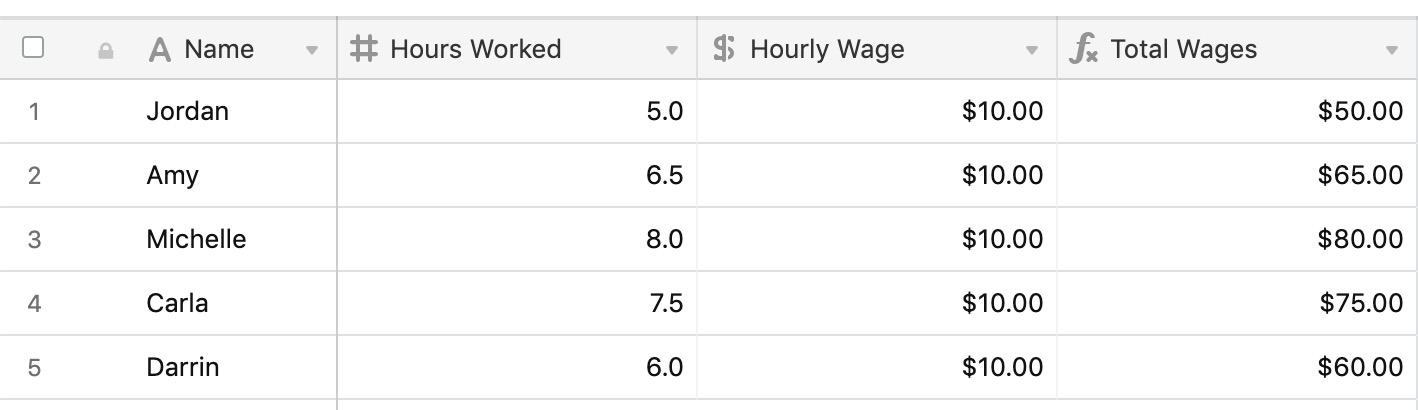In the {Total Wages} field, this formula is used to calculate the total wages for the day for each employee:

``````{Hours Worked} * {Hourly Wage}
``````

## Subtracting

You can accomplish similar calculations using the same formula structure. To find the difference between wages due and wages paid, you would use a formula like the following:

``````{Total Wages Due}-{Total Wages Paid}
``````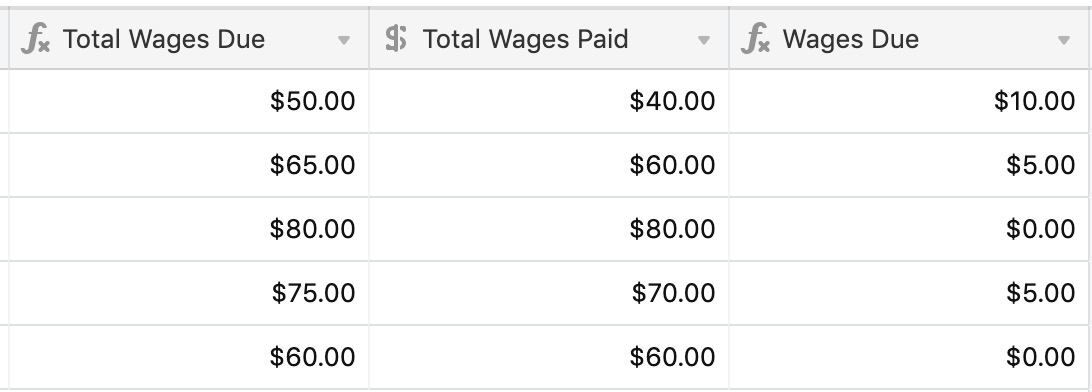## Dividing

In a different scenario, let's say that you're using Airtable to track your freelance project work. You quote a certain number of hours of service you will provide, and want to track your completion towards those hours.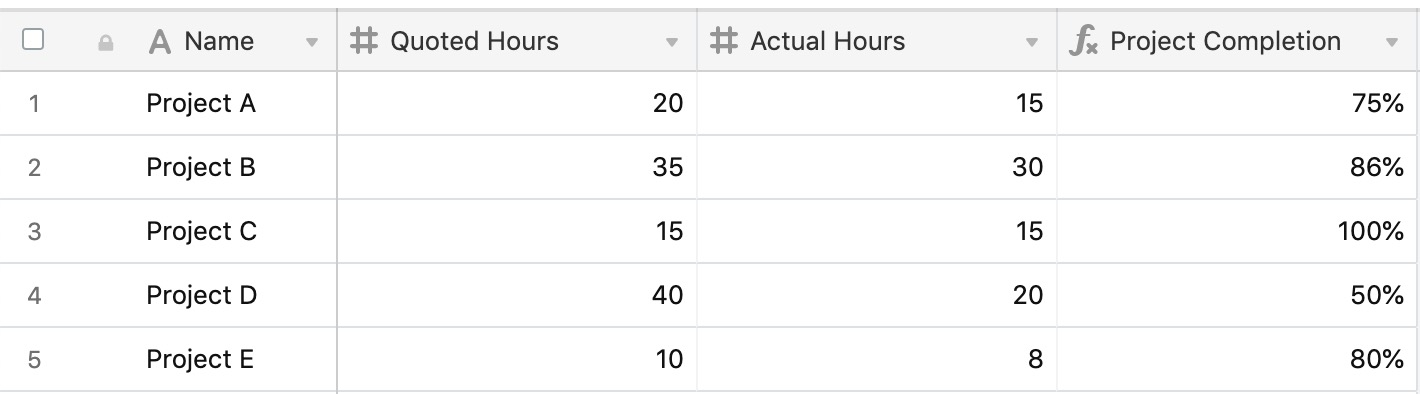In {Project Completion}, you would use a formula like the following to calculate your progress:

``````{Actual Hours} / {Quoted Hours}
``````

You can add multiple fields together by separating them with a + sign. In the example below, several fields are added together to find the final invoice total for a legal department.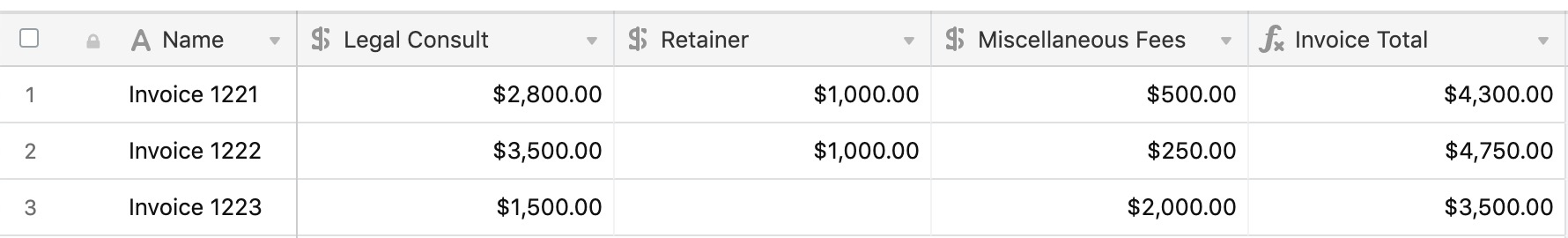The {Invoice Total}field uses the formula below:

``````{Legal Consult} + {Retainer} + {Miscellaneous Fees}
``````

## Add, subtract, multiply, and divide in one formula

You can run any number of different functions within the same formula. In the example below, several different factors play into finding the final amount to charge a client on a payment plan:

• Multiply {Consult: Hours} * {Consult {Rate}
• Add {Retainer} and {Miscellaneous fees}
• Subtract {Discounts}
• Divide everything by {Num. of Payments}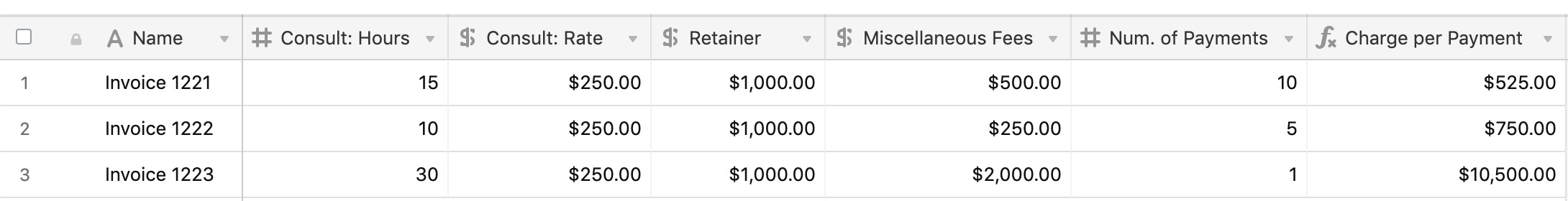All of these are built-in to a formula together in {Charge per Payment} using the formula below:

``````(
({Consult: Rate}*{Consult: Hours})
+ Retainer
+ {Miscellaneous Fees}
- {Discounts}
)
/{Num. of Payments}
``````

## Formatting a formula field

Note that for the examples above, the output of a formula can initially be a plain number string, and not have any formatting. You can apply formatting to a number field by double clicking the field name, selecting customize field type , and then formatting. Then, you can apply the following formatting to the output of your formula:

• Decimal
• Integer
• Currency
• Percent
• Duration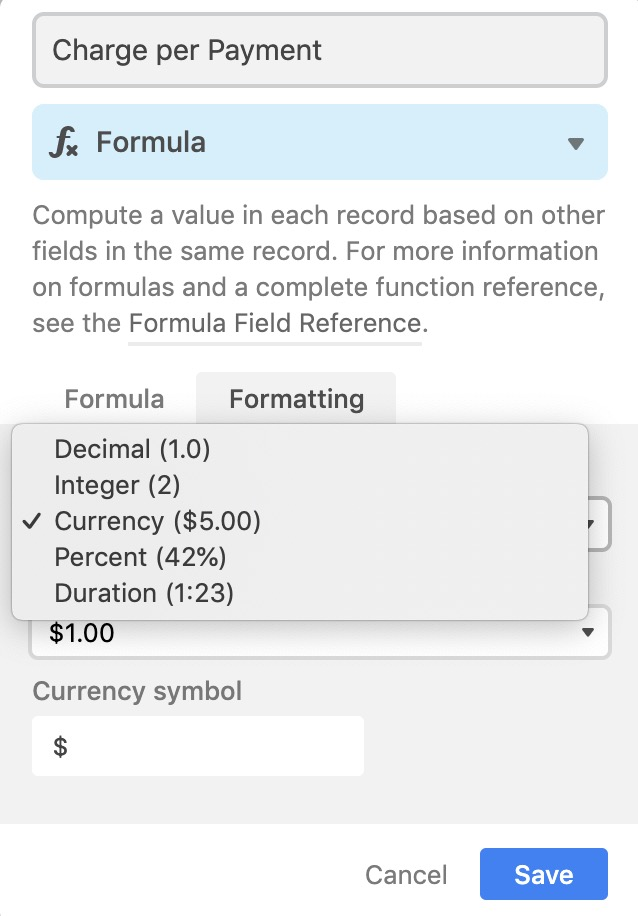## More on formulas

For more information on building formulas in Airtable see these related articles: